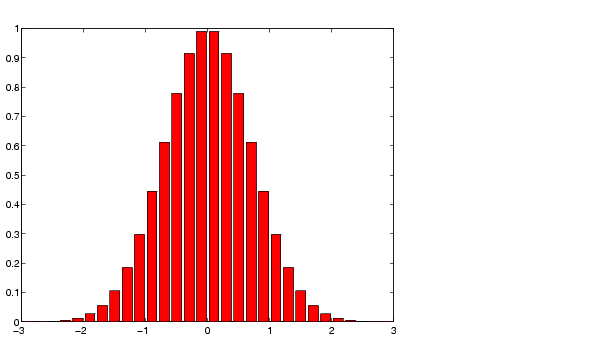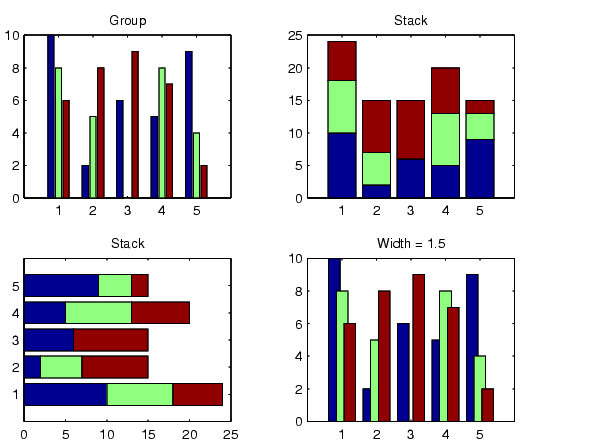MATLAB Function Referencebar, barh

Bar chart

Syntax

• bar(Y)
bar(x,Y)
bar(...,width)
bar(...,'style')
bar(...,LineSpec)
h = bar(...)

barh(...)
h = barh(...)

Description

A bar chart displays the values in a vector or matrix as horizontal or vertical bars.

bar(Y) draws one bar for each element in Y. If Y is a matrix, bar groups the bars produced by the elements in each row. The x-axis scale ranges from 1 to length(Y) when Y is a vector, and 1 to size(Y,1), which is the number of rows, when Y is a matrix.

bar(x,Y) draws a bar for each element in Y at locations specified in x, where x is a monotonically increasing vector defining the x-axis intervals for the vertical bars. If Y is a matrix, bar clusters the elements in the same row in Y at locations corresponding to an element in x.

bar(...,width) sets the relative bar width and controls the separation of bars within a group. The default width is 0.8, so if you do not specify x, the bars within a group have a slight separation. If width is 1, the bars within a group touch one another.

bar(...,'style') specifies the style of the bars. 'style' is 'grouped' or 'stacked'. 'group' is the default mode of display.

• 'grouped' displays n groups of m vertical bars, where n is the number of rows and m is the number of columns in Y. The group contains one bar per column in Y.
• 'stacked' displays one bar for each row in Y. The bar height is the sum of the elements in the row. Each bar is multi-colored, with colors corresponding to distinct elements and showing the relative contribution each row element makes to the total sum.

bar(...,LineSpec) displays all bars using the color specified by LineSpec.

h = bar(...) returns a vector of handles to patch graphics objects. bar creates one patch graphics object per column in Y.

barh(...), and h = barh(...) create horizontal bars. Y determines the bar length. The vector x is a monotonic vector defining the y-axis intervals for horizontal bars.

Examples

Plot a bell shaped curve:

• x = -2.9:0.2:2.9;
bar(x,exp(-x.*x))
colormap hsvCreate four subplots showing the effects of various bar arguments:

• Y = round(rand(5,3)*10);
subplot(2,2,1)
bar(Y,'group')
title 'Group'

• subplot(2,2,2)
bar(Y,'stack')
title 'Stack'

• subplot(2,2,3)
barh(Y,'stack')
title 'Stack'

• subplot(2,2,4)
bar(Y,1.5)
title 'Width = 1.5'balance bar3, bar3h